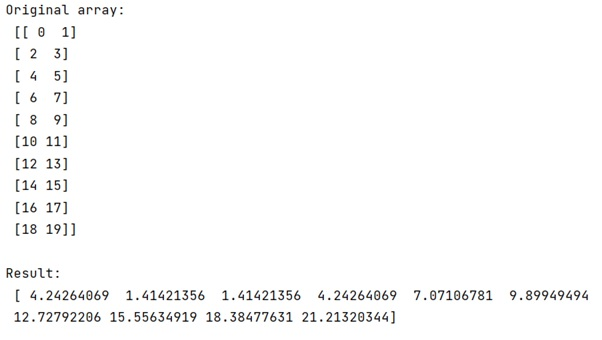# Euclidean distance calculation between matrices of row vectors

In this tutorial, we will learn how to calculate the Euclidean distance between matrices of row vectors in Python NumPy? By Pranit Sharma Last updated : May 05, 2023

Suppose that we have a NumPy array where each row is a vector and a single NumPy array. We need to calculate the Euclidean distance between all the points and this single point and store them in one NumPy array.

## How to calculate Euclidean distance between matrices of row vectors?

To calculate Euclidean distance between matrices of row vectors, there is an easier approach which is to use numpy.hypot(*(points - single_point).T) i.e., the transpose assumes that points are an Nx2 array, rather than a 2xN. If it is 2xN, we do not need the transpose.

In a simple context, we can first define a single point (row or a vector), then we can simply apply the distance formula to the array and the single point.

Let us understand with the help of an example,

## Python program to calculate Euclidean distance calculation between matrices of row vectors

```# Import numpy
import numpy as np

# Creating an array
arr = np.arange(20).reshape((10,2))

# Single point
sp = [3,4]

# Display original array
print("Original array:\n",arr,"\n")

# Finding distance
dis = (arr - sp)**2
dis = np.sum(dis, axis=1)
dis = np.sqrt(dis)

# Display result
print("Result:\n",dis)
```

### Output